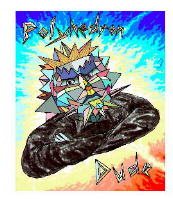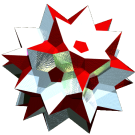Acronym gassid Name great snub dodecahedron,compound of 6 pap `© ` Circumradius sqrt[(5+sqrt(5))/8] = 0.951057 Vertex figure [33,5] Dihedral angles (at margins) between {3} and {3}:   arccos(-sqrt(5)/3) = 138.189685° between {3} and {5}:   arccos(-sqrt[(5-2 sqrt(5))/15]) = 100.812317° ExternallinksIt can be obtained by inscribing 6 pap into an ike (in each of the axial orientations), and then rotating each by 36° around its axis, so that the pentagons each position in the dual orientation than before.

A double cover of gassid occurs as special case of gadsid.

Incidence matrix

```  60 |  2  2 |  3  1 || 1
-----+-------+-------++--
2 | 60  * |  1  1 || 1
2 |  * 60 |  2  0 || 1
-----+-------+-------++--
3 |  1  2 | 60  * || 1
5 |  5  0 |  * 12 || 1
-----+-------+-------++--
♦ 10 | 10 10 | 10  2 || 6
```